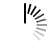## Array program in LISP

```(setf x (make-array '(3 3):initial-contents'((1 2 3)(4 5 6)(7 8 9))))
(write x)

(defun afisare (i j g h)
(print (aref x i j))
(print (aref x g h))
)

(afisare 0 1 0 2)

(setq myarray (make-array '(3 2 3)
:initial-contents
'(((a b c) (1 2 3))
((d e f) (4 5 6))
((g h i) (7 8 9))
))
)
(setq array2 (make-array '(3 2) :displaced-to myarray :displaced-index-offset 5))
(write myarray)
(terpri)
(write array2)

(setf (get 'student 'Nume) 'Dan)
(setf (get 'student 'Varsta) 21)
(setf (get 'student 'An) 3)
(setf (get 'student 'cnp) 1970506350036)

(terpri)
(write (symbol-plist 'student))

(defun averagenum (n1 n2 n3)
(/ ( + n1 n2 n3) 3)
)
(write(averagenum 5 10 6))```

## Getting Average of Numbers using LISP

```(setf x (make-array '(3 3)
:initial-contents '((1 2 3 ) (4 5 6) (7 8 9)))
)
(write x)

(defun afisare (i j)
(print (aref x i j))
)

(afisare 0 1)

(setq a (make-array '(2 2):displaced-to x :displaced-index-offset 5 ))
(write a)

(setf (get 'student 'age) 43)
(setf (get 'student 'cnp) '123456789)
(setf (get 'student 'anstudiu) 3)
(setf (get 'student 'ioan)  1)

(terpri)
(write (symbol-plist 'student))

(defun avrege()
(terpri)
(princ "Enter number 1: ")
(write x)
(princ "enter number 2")

(write y)
(princ "enter number 3")

(write z)
(setq avrege (/ (+ x y z) 3))
(princ "avrege: ")
(write avrege)
)

(avrege)```

## Function to take average of numbers using LISP

```(print"Lucrarea 3")
(terpri)

(setf a
(make-array '(3 3)
:initial-contents '((1 2 3) (4 5 6) (7 8 9))
)
)
(write a)
(terpri)

(defun afisare(i j)
(print (aref a i j)))
(afisare 1 2)
(terpri)

(setq a2 (make-array '(2 2) :displaced-to a :displaced-index-offset 5))
(write a2)
(terpri)

(setf (get 'Student'nume) 'Mihai)
(setf (get 'Student'varsta)30)
(setf (get 'Student'AnStudiu)3)
(setf (get 'Student'CNP)18403123456)
(write (symbol-plist 'Student))
(terpri)

(defun averagesum(n1 n2 n3 n4 n5 n6 n7 n8 n9)
(/(+ n1 n2 n3 n4 n5 n6 n7 n8 n9)9))
(write(averagesum 5 6 7 5  5 4 7 8 9))```

## Using Array of Numbers in LISP

```(setf x (make-array '(3 3)   :initial-contents '((1 2 3 ) (4 5 6) (7 8 9)))
)
(write x)
```

## (- 10 (* 20 35 )(* 3.5 (+ 15 65)))

```;(write-line "Hello World")
(- 10 (* 20 35 ) (* 3.5 (+ 15 65)))```

## Toms 60 harc 5

```(defun f (L)
(if(NULL L) nil
(if(> (car L) 10) (f (cdr L))
(cons (* (car L) 2) (f (cdr L)))))
)
(write (f '(15 5 15 2 11)))```

## Toms 60 harc 4

```(defun fact (y)
(if(= y 0) 1
(if(= y -1) 1
(* y (fact (- y 2)))))
)

(defun sum (x)
( if(= 0 x) 0
(+ (sum (- x 1)) (* x (* x x))))
)

(defun f (x y)
(/ (sum x) (fact y))
)
(write (f 10 10))```

## Execute LISP Online

```(defun f (x y)
(if (= y 0)
(+ x 1)
(* (f x (- y 1)) (+ (+ x 1) y)))
)
(defun g (x y)
(+ (f x y) y)
)
(write(g 10 10))```

## Execute LISP Online

```(defun f (n &optional (k 0) (c 1)  )
(if (>= c n)
k
( f  n (+ k 1) (* c 2)))
)
(+ 1 0)
(write(f 4  ))```

## Execute LISP Online

```(defun f (n &optional (k 0) &optional (c 1)  )
(if (>= c n)
k
( f  n (+ k 1) (* c 2)))
)
(+ 1 0)
(write(f 4 0 ))```